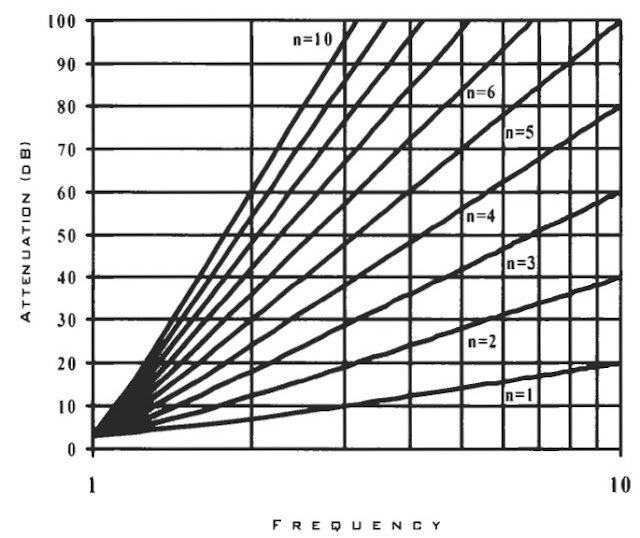# Butterworth Passive Low Pass Filter Design

Here Butterworth passive LPF design is illustrated with worked out example. The required filter specification is 60dB attenuation with cutoff frequency of 4MHz. Passive filter is build using combination of resistors, inductors and capacitors. Here inductor and capacitor in ladder network is used to construct the filter.

The step to design Butterworth filter is to first set desired attenuation and calculate the order of the filter with desired cutoff frequency of interest. The order can be calculated using formula or a graph can be used. After knowing the order of the filter a normalized filter is constructed. Later on  the normalized circuit is de-normalized using component and frequency scaling to obtain the final filter.

### Butterworth frequency response

In terms of frequency response this type of filter has a flat response in the passband among all filters. The frequency response graph of Butterworth filter is shown below.

The formula for attenuation for Butterworth is as follows,

$$A(dB)=10 log_{10}[1+(\frac{w}{w_c})^{2n}]$$

where, $$A(dB)$$ is attenuation in dB

$$w$$ is the angular frequency in radian

$$w_c$$ is the cutoff frequency in radian

$$n$$ is order of the filter

For example, with cutoff frequency f=4MHz and $$w=2 \pi f$$,order of filter, n=5 the attenuation is 60dB.

$$A(dB)=10 log[1+4^{2\times 5}]$$

Also the Butterworth transfer function is given below,

$$H(w) = \frac{1}{\sqrt{1+(\frac{w}{w_c})^{2n}}}$$

where n is the order of the filter

The following graph shows the attenuation variation(increase) with frequency and order of the filter which is obtained using the above attenuation equation.Passive Butterworth can be designed using inductor and capacitor in ladder network topology. The first component in the ladder can be either capacitor or inductor.

The following shows 5th order passive Butterworth LPF in which the first component is a capacitor.

In the following the first component is an inductor.

Conditions:

1. $$R_L >> R_S$$

If the load resistance is much greater than source resistance(say 10 times) then,

- the load is considered to be have infinite impedance or is open circuited

- the last component must be shunt capacitor

- odd order filter starts with shunt capacitor

- even order filter starts with series inductor

2. $$R_L << R_S$$

If the load resistance is much lower than the source resistance(say by 10 times) then,

- the load is considered to have zero impedance or is short circuited

- the last component must be a series inductor

- odd order filter starts with series inductor

- even order filter starts with shut capacitor

Butterworth table for Rs=0 and Rs=infinity

3. $$R_L \simeq R_S$$

When load resistance is close to source resistance then we use the following Butterworth normalized component value table.

Normalized Fifth order Butterworth LPF

We need two scaling to get the proper values of the components. They are component scaling and frequency scaling.

Component Scaling

To de-normalize normalized LPF circuit we need proper transformation of the component values. If the load resistance is say 50Ohm which is more practical resistance we need proper transformation of the component values. The impedance of inductor is proportional to the inductance and the impedance of a capacitor is inversely proportional to the capacitance. Hence for proper transform, we multiple each inductor component with 50 and divide each capacitor component by 50 to get the component scaled filter.

Frequency Scaling

The frequency scaling factor is $$2 \pi f$$. The cutoff frequency(fc) is 4MHz. Therefore the frequency scaling value is,

$$2 \pi f = 2 \pi \times 4MHz = 25.133 \times 10^6$$

Formula

The component scaling and frequency scaling can be done using the following transformation formula.

$$L = \frac{R L_n}{2\pi f_c}$$

$$C = \frac{C_n}{2\pi f_c R}$$

where, $$L_n$$ and $$C_n$$ are normalized low pass component values.

Dividing the component values in the component scaled circuit we get the following circuit.

In this way one can design Butterworth passive low pass filter. A second order LPF design using only capacitor and inductor is like LC filter(see Second Order LC Low Pass Filter Calculator).